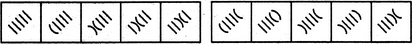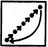# Non Verbal Reasoning - Series - Discussion

Discussion Forum : Series - Section 1 (Q.No. 10)
Directions to Solve

Each of the following questions consists of five figures marked A, B, C, D and E called the Problem Figures followed by five other figures marked 1, 2, 3, 4 and 5 called the Answer Figures. Select a figure from amongst the Answer Figures which will continue the same series as established by the five Problem Figures.

10.

Select a figure from amongst the Answer Figures which will continue the same series as established by the five Problem Figures.(A)     (B)     (C)     (D)     (E)       (1)     (2)     (3)     (4)     (5)
1
2
3
4
5
Explanation:
In the first step, the lowermost line segment is converted into a curve. In the second step, the second line segment also gets converted into a curve and the (existing curve is inverted. In each subsequent step, all the elements (line segments and curves) move in the sequenceDiscussion:
4 comments Page 1 of 1.

Omkar said:   7 months ago